# 032616 week3 impulse_and_momentum

Faculty at University of Phoenix
26 de Mar de 2016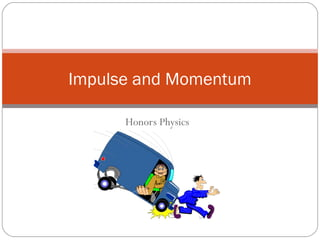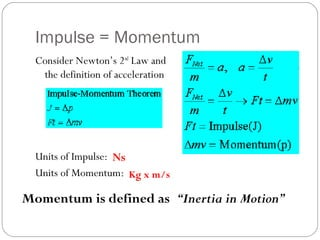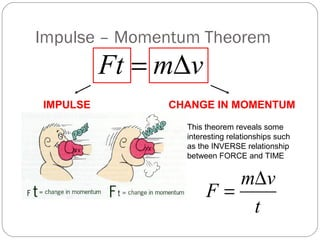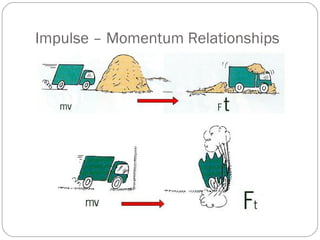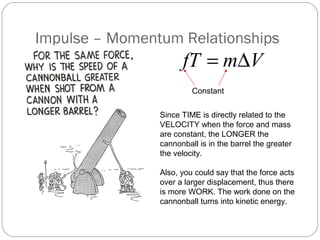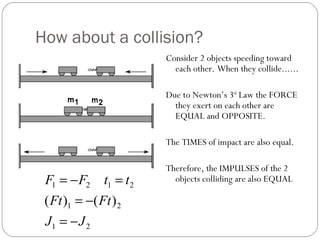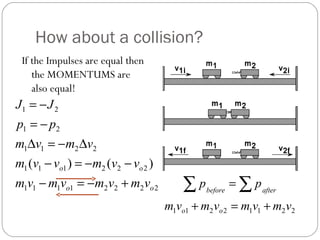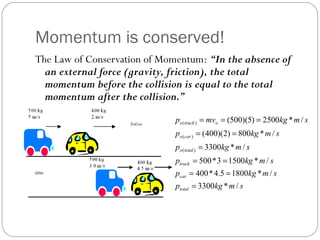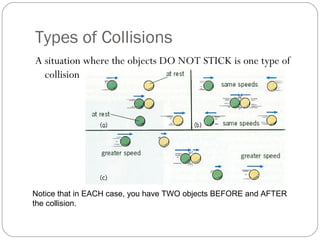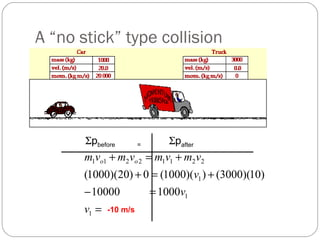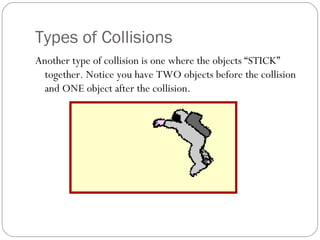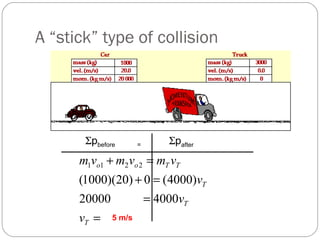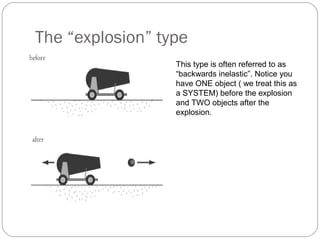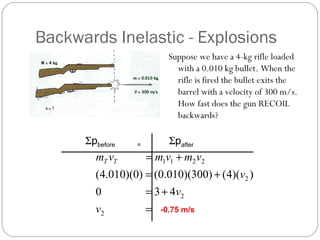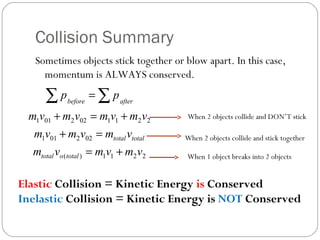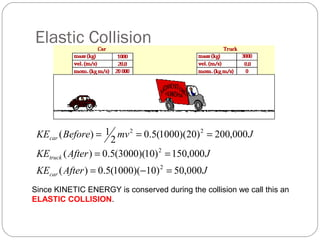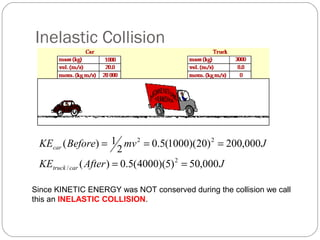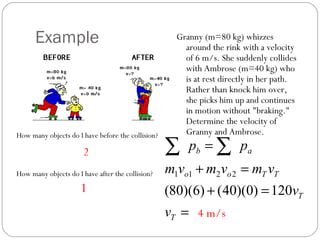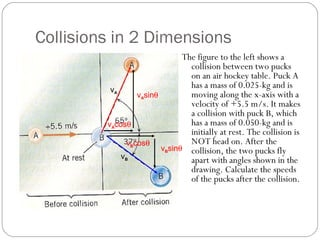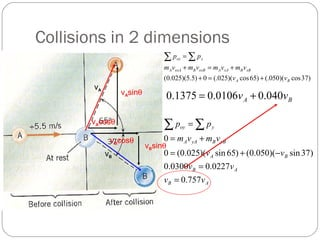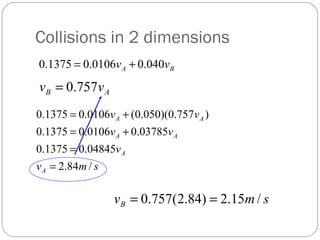1 de 21

### 032616 week3 impulse_and_momentum

1. Honors Physics Impulse and Momentum
2. Impulse = Momentum Consider Newton’s 2nd Law and the definition of acceleration Units of Impulse: Units of Momentum: Momentum is defined as “Inertia in Motion” Ns Kg x m/s
3. Impulse – Momentum Theorem vmFt ∆= IMPULSE CHANGE IN MOMENTUM This theorem reveals some interesting relationships such as the INVERSE relationship between FORCE and TIME t vm F ∆ =
4. Impulse – Momentum Relationships
5. Impulse – Momentum Relationships VmfT ∆= Constant Since TIME is directly related to the VELOCITY when the force and mass are constant, the LONGER the cannonball is in the barrel the greater the velocity. Also, you could say that the force acts over a larger displacement, thus there is more WORK. The work done on the cannonball turns into kinetic energy.
6. How about a collision? Consider 2 objects speeding toward each other. When they collide...... Due to Newton’s 3rd Law the FORCE they exert on each other are EQUAL and OPPOSITE. The TIMES of impact are also equal. Therefore, the IMPULSES of the 2 objects colliding are also EQUAL 21 21 2121 )()( JJ FtFt ttFF −= −= =−=
7. How about a collision? If the Impulses are equal then the MOMENTUMS are also equal! 22221111 222111 2211 21 21 )()( oo oo vmvmvmvm vvmvvm vmvm pp JJ +−=− −−=− ∆−=∆ −= −= 22112211 vmvmvmvm pp oo afterbefore +=+ = ∑∑
8. Momentum is conserved! The Law of Conservation of Momentum: “In the absence of an external force (gravity, friction), the total momentum before the collision is equal to the total momentum after the collision.” smkgp smkgp smkgp smkgp smkgp smkgmvp total car truck totalo caro otrucko /*3300 /*18005.4*400 /*15003*500 /*3300 /*800)2)(400( /*2500)5)(500( )( )( )( = == == = == ===
9. Types of Collisions A situation where the objects DO NOT STICK is one type of collision Notice that in EACH case, you have TWO objects BEFORE and AFTER the collision.
10. A “no stick” type collision Σpbefore = Σpafter = =− +=+ +=+ 1 1 1 22112211 100010000 )10)(3000())(1000(0)20)(1000( v v v vmvmvmvm oo -10 m/s
11. Types of Collisions Another type of collision is one where the objects “STICK” together. Notice you have TWO objects before the collision and ONE object after the collision.
12. A “stick” type of collision Σpbefore = Σpafter = = =+ =+ T T T TToo v v v vmvmvm 400020000 )4000(0)20)(1000( 2211 5 m/s
13. The “explosion” type This type is often referred to as “backwards inelastic”. Notice you have ONE object ( we treat this as a SYSTEM) before the explosion and TWO objects after the explosion.
14. Backwards Inelastic - Explosions Suppose we have a 4-kg rifle loaded with a 0.010 kg bullet. When the rifle is fired the bullet exits the barrel with a velocity of 300 m/s. How fast does the gun RECOIL backwards? Σpbefore = Σpafter = += += += 2 2 2 2211 430 ))(4()300)(010.0()0)(010.4( v v v vmvmvm TT -0.75 m/s
15. Collision Summary Sometimes objects stick together or blow apart. In this case, momentum is ALWAYS conserved. 2211)( 022011 2211022011 vmvmvm vmvmvm vmvmvmvm pp totalototal totaltotal afterbefore += =+ +=+ = ∑∑ When 2 objects collide and DON’T stick When 2 objects collide and stick together When 1 object breaks into 2 objects Elastic Collision = Kinetic Energy is Conserved Inelastic Collision = Kinetic Energy is NOT Conserved
16. Elastic Collision JAfterKE JAfterKE JmvBeforeKE car truck car 000,50)10)(1000(5.0)( 000,150)10)(3000(5.0)( 000,200)20)(1000(5.0 2 1)( 2 2 22 =−= == === Since KINETIC ENERGY is conserved during the collision we call this an ELASTIC COLLISION.
17. Inelastic Collision JAfterKE JmvBeforeKE cartruck car 000,50)5)(4000(5.0)( 000,200)20)(1000(5.0 2 1)( 2 / 22 == === Since KINETIC ENERGY was NOT conserved during the collision we call this an INELASTIC COLLISION.
18. Example Granny (m=80 kg) whizzes around the rink with a velocity of 6 m/s. She suddenly collides with Ambrose (m=40 kg) who is at rest directly in her path. Rather than knock him over, she picks him up and continues in motion without "braking." Determine the velocity of Granny and Ambrose.How many objects do I have before the collision? How many objects do I have after the collision? 2 1 = =+ =+ = ∑∑ T T TToo ab v v vmvmvm pp 120)0)(40()6)(80( 2211 4 m/s
19. Collisions in 2 Dimensions The figure to the left shows a collision between two pucks on an air hockey table. Puck A has a mass of 0.025-kg and is moving along the x-axis with a velocity of +5.5 m/s. It makes a collision with puck B, which has a mass of 0.050-kg and is initially at rest. The collision is NOT head on. After the collision, the two pucks fly apart with angles shown in the drawing. Calculate the speeds of the pucks after the collision. vA vB vAcosθ vAsinθ vBcosθ vBsinθ
20. Collisions in 2 dimensions vA vB vAcosθ vAsinθ vBcosθ vBsinθ )37cos)(050(.)65cos)(025(.0)5.5)(025.0( BA xBBxAAoxBBoxAA xox vv vmvmvmvm pp +=+ +=+ =∑ ∑ BA vv 040.00106.01375.0 += AB AB BA yBByAA yoy vv vv vv vmvm pp 757.0 0227.00300.0 )37sin)(050.0()65sin)(025.0(0 0 = = −+= += =∑ ∑
21. Collisions in 2 dimensions AB vv 757.0= smv v vv vv A A AA AA /84.2 04845.01375.0 03785.00106.01375.0 )757.0)(050.0(0106.01375.0 = = += += BA vv 040.00106.01375.0 += smvB /15.2)84.2(757.0 ==